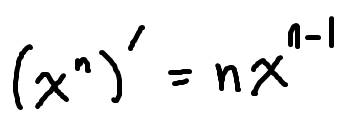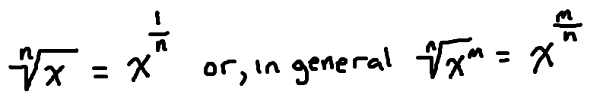﻿ The power rule for derivatives - MathBootCamps

# The power rule for derivatives

Usually the first shortcut rule you study for finding derivatives is the power rule. The reason is that it is a simple rule to remember and it applies to all different kinds of functions. For a number n, the power rule states:Let’s start with some really easy examples to see it in action.

### Example

Find the derivative of each function.

$$\text{(a) } x^4$$

$$\text{(b) } x^{10}$$

$$\text{(c) } x^{546}$$

### Solution

For each of these, you can simply apply the power rule without any algebra at all. This means that you should bring the exponent out front, and then subtract 1 from the exponent.

$$\text{(a) } \left(x^4\right)^{\prime} = 4x^3$$

$$\text{(b) } \left(x^{10}\right)^{\prime} = 10x^9$$

$$\text{(c) } \left(x^{546}\right)^{\prime} = 546x^{545}$$

As you can see, it is all about remembering the pattern. Now, we will see how this pattern can be applied to more complicated examples.

## Derivatives of polynomial functions

Recall that the derivative of a constant is always zero. So, the derivative of 5 is 0 while the derivative of 2,000 is also 0. Further, you can break the derivative up over addition/subtraction and multiplication by constants. Combining these ideas with the power rule allows us to use it for finding the derivative of any polynomial.

### Example

Find the derivative of the function.
$$y = 2x^4 – 5x^2 + 1$$

### Solution

With a little bit of practice, you will probably be able to write the derivative of this function down without thinking. Since it is our first example though, let’s write out every step. In the first step, we will break the derivative up over the addition and subtraction.

\begin{align} y^{\prime} &= \left(2x^4 – 5x^2 + 1\right)^{\prime}\\ &= \left(2x^4\right)^{\prime} – \left(5x^2\right)^{\prime} + \left(1\right)^{\prime}\end{align}

Now, factor out the coefficients:

$$= 2\left(x^4\right)^{\prime} – 5\left(x^2\right)^{\prime} + \left(1\right)^{\prime}$$

Apply the power rule for derivatives and the fact that the derivative of a constant is zero:

$$= 2\left(4x^3\right) – 5\left(2x^1\right) + \left(0\right)$$

Notice that once we applied the derivative rule, the prime went away. The correct notation keeps this until you apply a derivative rule. Now all we need to do is simplify to get our final answer.

$$= \boxed{8x^3 – 10x}$$

Let’s look at one more example without so much explanation to distract us.

### Example

Find the derivative of the function.
$$y = 5x^3 – 3x^2 + 10x – 8$$

### Solution

Apply the power rule, the rule for constants, and then simplify. Note that if $$x$$ doesn’t have an exponent written, it is assumed to be 1.

\begin{align} y^{\prime} &= \left(5x^3 – 3x^2 + 10x – 8\right)^{\prime}\\ &= 5\left(3x^2\right) – 3\left(2x^1\right) + 10\left(x^0\right)- 0\end{align}

Since $$x$$ was by itself, its derivative is $$1x^0$$. Normally, this isn’t written out however. Just remember that anything (other than zero) to the zero power is 1. So, $$10\left(x^0\right) = 10(1) = 10$$. Therefore, we can write the final answer as:

$$= \boxed{15x^2 – 6x + 10}$$

You may think this is all you can really do with the power rule. However, a couple of old algebra facts can help us apply this to a wider range of functions. We will look at two of those instances below.

## Derivatives of functions with negative exponents

The power rule applies whether the exponent is positive or negative. But sometimes, a function that doesn’t have any exponents may be able to be rewritten so that it does, by using negative exponents. If this is the case, then we can apply the power rule to find the derivative. The main property we will use is:Let’s see what we can do with this property using an example!

### Example

Find the derivative of the function.
$$y = \dfrac{2}{x^4} – \dfrac{1}{x^2}$$

### Solution

Applying the rule for negative exponents, we can rewrite this function as:

$$y = \dfrac{2}{x^4} – \dfrac{1}{x^2} = 2x^{-4} – x^{-2}$$

Now that this is written with exponents, we can apply the power rule:

\begin{align} y^{\prime} &= \left(2x^{-4} – x^{-2}\right)^{\prime}\\ &= 2\left(-4x^{-4-1}\right) – \left(-2x^{-2-1}\right)\end{align}

Simplify to find the final answer:

\begin{align} &= -8x^{-5} +2x^{-3}\\ &= \boxed{-\dfrac{8}{x^{5}} + \dfrac{2}{x^{3}}}\end{align}

In the last step, notice that only the terms with the negative exponent were moved to the bottom of the fraction. The 8 didn’t have a negative exponent, so it stayed.

## Derivatives of functions with radicals (square roots and other roots)

Another useful property from algebra is the following.Using this rule, we can take a function written with a root and find its derivative using the power rule.

### Example

Find the derivative of the function.
$$y = 4\sqrt{x} – 6\sqrt{x^2}$$

### Solution

First, rewrite the function using algebra:

$$y = 4\sqrt{x} – 6\sqrt{x^2} = 4x^{\frac{1}{2}} – 6x^{\frac{2}{3}}$$

Now apply the power rule:

\begin{align} y^{\prime} &= \left(4x^{\frac{1}{2}} – 6x^{\frac{2}{3}}\right)^{\prime}\\ &= 4\left(\dfrac{1}{2}x^{\frac{1}{2}-1}\right) – 6\left(\dfrac{2}{3}x^{\frac{2}{3}-1}\right)\end{align}

Simplify to get the final answer, remembering the rule for negative exponents:

\begin{align} &= 2x^{-\frac{1}{2}} – 4x^{-\frac{1}{3}}\\ &= \dfrac{2}{x^{\frac{1}{2}}} – \dfrac{4}{x^{\frac{1}{3}}}\\ &= \dfrac{2}{\sqrt{x}} – \dfrac{4}{\sqrt{x}}\end{align}

In many classes, either of the last two lines can be written as your final answer. They are equivalent. However, your teacher or professor may have a preference, so always ask!

## Summary

As a student studying calculus, you want the power rule to be second nature. It’ll be applied not only like this – on its own – but also as part of other rules such as the chain rule, the quotient rule, and the product rule. The better you understand it, the more you can focus on those more complicated ideas.

## Continue studying derivatives

Previous: The derivative of a constant

Next: The product rule## Subscribe to our Newsletter!

We are always posting new free lessons and adding more study guides, calculator guides, and problem packs.

Sign up to get occasional emails (once every couple or three weeks) letting you know what's new!